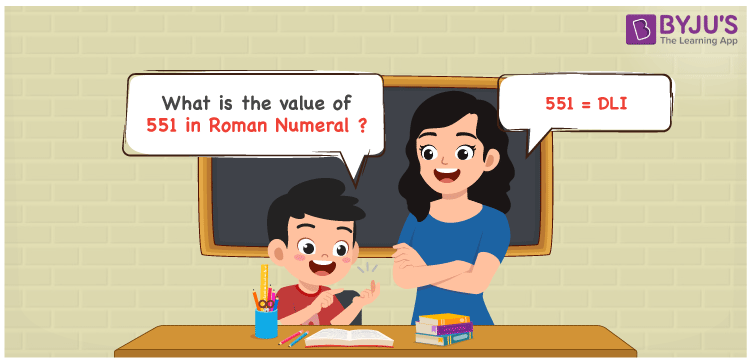# 551 in Roman Numerals

551 in Roman Numerals is DLI. To represent any number in Roman numerals, the 7 primary symbols are used with certain rules. Refer to the rules of Roman numerals. DLI follows the addition rule to get the number 551. Here D equals 500, L equals 50 and I equals 1.

 Number Roman Numeral 551 DLI

## How to Write 551 in Roman Numerals?To convert 551 in Roman Numerals, we need to split the number according to the available symbols. i,e I, V, X, L, C, D, and M.

The simple way to split 551 will be 500 + 50 + 1. Since there are primary symbols for these three numbers, 551 can be represented as DLI.

Let us understand it numerically

551 = 500 + 50 + 1

551 = D + L + I

551 = DLI

## Video Lesson on Roman Numerals## Frequently Asked Questions on 551 in Roman Numerals

Q1

### How to write the number 551 in Roman Numerals?

551 in Roman Numerals is written as DLI.
Q2

### Do the Roman numerals M and DD represent the number 1000?

No. Only the Roman numeral M represents 1000. D should not be repeated and hence DD is an invalid Roman numeral.
Q3

### How to read the Roman numeral DLI in English

The Roman Numeral DLI represents 551 and it is read as Five Hundred and Fifty One.